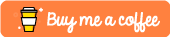# Editing Images Via A Prompt With Python And Pytorch

## Introduction

Hello! 😃 In this tutorial I will show you how you can use a pre-trained machine learning model to modify an image based on the user's input prompt. The model uses an image editing technique called "instruct-pix2pix" and is implemented in Python using the PyTorch module.

Well then let's get started. 😎

## Requirements

• Basic knowledge of Python
• A decent spec computer

## Creating The Virtual Environment

First we need to create a virtual Python environment for the project. Open up the terminal and run the following command in the project's root directory:

``````python3 -m venv env
``````

Next we need to activate the environment which can be done via the following command:

``````source venv/bin/activate
``````

Next we need to install the dependencies. 💫

## Installing The Dependencies

To install the dependencies, open up a file called "requirements.txt" and add the following modules:

``````diffusers
transformers
accelerate
ipython
``````

Next run the following command:

``````pip install -r requirements.txt
``````

Now we can finally start coding! ☺️

## Coding The Application

Next we can finally start writing the source code, open up a file called "main.py" and import the following:

``````import PIL
import torch
from diffusers import StableDiffusionInstructPix2PixPipeline
import argparse
``````

Next, we need to initialize some constant variables:

``````MODEL_ID = "timbrooks/instruct-pix2pix"
#PIPE = StableDiffusionInstructPix2PixPipeline.from_pretrained(MODEL_ID, torch_dtype=torch.float16).to("cuda")
PIPE = StableDiffusionInstructPix2PixPipeline.from_pretrained(MODEL_ID).to("cpu")
``````

Here we define the model to use. (in this case instruct-pix2pix) The repo for this can be found here: https://github.com/timothybrooks/instruct-pix2pix

We also initialize the pipeline, if your machine has a decent amount of GPU VRAM I highly recommend using the commented out line. My machine isn't that great of spec so I opted to use the CPU over GPU. 🥺

Next we will create the main method:

``````def main(prompt, imagePath):
image = PIL.Image.open(imagePath)

images = PIPE(prompt, image = image, num_inference_steps = 30, image_guidance_scale = 1.5, guidance_scale = 7).images

new_image = PIL.Image.new("RGB", (image.width * 2, image.height))
new_image.paste(image, (0, 0))
new_image.paste(images, (image.width, 0))

new_image.save("output.png")
``````

What this method does is open the image file from the image path that was passed to it, which will then use the pre-trained model to modify the image based on the provided prompt.

Finally we combine both the original image and the new image side by side so that we can compare them and then save the image to a file called "output.png".

Next we add the following in order to call the main method:

``````if __name__ == "__main__":
ap = argparse.ArgumentParser()
ap.add_argument("-i", "--image", required = True, help = "Path to image file")
ap.add_argument("-p", "--prompt", required = True, help = "Prompt for image editing")

args = vars(ap.parse_args())

main(args["prompt"], args["image"])
``````

All the above does is take an image file path and a prompt from the command line and then passes them both to the main method.

All done! 😄

You can try the program with the following command:

``````python main.py -i [path to image file] -p [prompt]
``````

Depending on the spec of your machine you may need to wait a while for the image to be processed. If you run into any out of memory issues try decreasing the size of the image or the amount of num_inference_steps. 👀

## Conclusion

Here I have shown how to edit images with Python, PyTorch and by using a pre-trained model.

I hope you learned something from this tutorial as much as I did writing it. 😆

You can find the source code for the tutorial via my Github: https://github.com/ethand91/python-pytorch-image-editor

As always happy coding! 😎

Like me work? I post about a variety of topics, if you would like to see more please like and follow me. Also I love coffee.If you are looking to learn Algorithm Patterns to ace the coding interview I recommend the following course